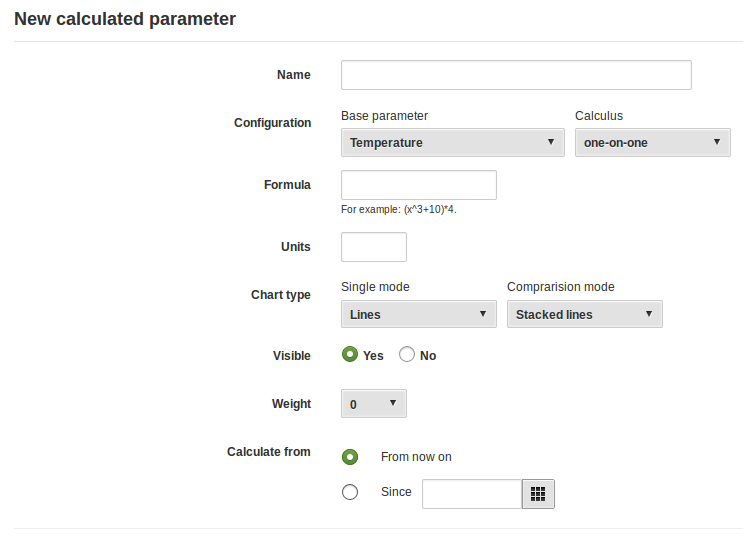# Parameters

In this article, we will explain how to create a calculated and a derived parameter, as well as how to create the hourly reactive energy parameter:

### 1. Create a calculated parameter

1. To create a new calculated parameter, go to "Parameters" screen on "Settings" menu.2. If you want to create a new calculated parameter, go to the "Calculated Parameters" and click on "New calculated parameter". The options are identical to those of the basic parameters except set point. We present the most relevant:
• Introduce a Name for the new parameter.
• Select a Base parameter. The calculated parameter will be created from a base parameter.
• If you don't have any data in the base parameter, the calculated parameter will not have any data either.
• Then, select one of the supported calculus [one-by-one, cumulative, average, max, min] and sampling frequency [ hourly, daily, weekly, monthly].

-  One-by-one: Formula calculation will be applied.
-  Cumulative: a basic parameter integral will be done.
-  Average: Geometric average calculation will be performed, based on the period selected in sampling rate field.
-  max / min: maximum or minimum points for the selected period based on the sampling rate will be done.

• Formula: This field allows you to add an additional formula to be performed on the calculated parameter.
• Units: units from the formula
• Weight: indicates the level of importance of the parameter, in order to list the parameters by the level of priority
• Chart type: which type of chart you want to generate (columns or rows). Also, you have to indicate if you make it visible or not.
• Calculate from: Select date from which parameter has to be calculated. Since option we go back in time and calculate the new variable from that moment.
• Calculated parameters can be Edited, Deleted or Recalculated.You can view and analyse your new parameters in the Analysis screen, in the "By Device" or Advanced Analytics menus or in "By parameter" Widgets.

Calculated parameters are free!

### 2. Create Derived parameters

Following this manual you will be able to convert a parameter into another one, simply doing a bypass or converting it with different correction factors.

This is useful when the gateway does not send data into DEXCell Energy Manager with the correct parameter identifiers or when you are doing Gas Metering.
1. Log in as a superadmin into your DEXCell Energy Manager account.
2. Go to "Settings" and click on "Gateways"3. Select a gateway and click on the "devices" tab.4. Edit the device you want to create a derived parameter from5.  From any device, you will be able to derive as many different parameters as you want. For example, from a Gas Massflow (m3), you can get Gas normalised massflow (Nm3) and Gas Energy (kWh).
6. On the form, focus on [Optional] Derived parameters7.   Now just select a "Base Parameter", a conversion formula if needed and the "Resultant parameter"
• Example 1: Bypass parameter - If you just need to bypass real-time data, for example from Parameter 503 to any other, use this configuration:• Example 2: Derived parameter - If you need to do some operations during the bypass, use the following configuration (with your own correct factors):1. Then save and that's all!

### 3. Creating the Hourly Reactive Energy Parameter

1. Go to the "Parameters" screen in the "Settings" menu.2. Go to "Calculated Parameters" tab, and click on "New calculated parameter" button
3. Write a name on "Name" field. i.e. Hourly Reactive Energy
4. From base parameter, select: Reactive energy.
5. On calculus, select "Cumulative"
6. On Sampling rate, select "Hourly"
7. Formula field can rest in blank
8. In units, write your according unit. In this case, energy comes in kWh, so reactive energy is kVArh.
9. Coming parameters can be let as shown in below image.
10. In calculate from field, select starting date where you would like to have the parameter available.1 out of 1 found this helpful

### Comments

0 comments

Please sign in to leave a comment.